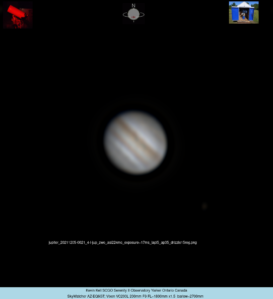seeing = 1 = DISGUSTING
Initial image full res wide view overexposed to confirm moon orientation no barlow.
Ganymede further out on left, Europa closer in on right
then to x1.5 barlow for this image and a smaller Region Of Interest (ROI).
Still looking for a powered USB hub to help with the USB powered motorized remote focuser… arriving any day now.
Still may need a tad bit of tiny optics collimation.

FireCapture v2.6 Settings
————————————
Camera=ZWO ASI224MC
Filter=L
Profile=Jupiter
Diameter=37.87″
Magnitude=-2.27
CMI=129.2° CMII=200.6° CMIII=52.5° (during mid of capture)
FocalLength=2900mm
Resolution=0.26″
Filename=2021-12-05-0021_4-L-Jup_ZWO ASI224MC_Exposure=16.9ms.ser
Date=051221
Start=001954.054
Mid=002124.055
End=002254.057
Start(UT)=001954.054
Mid(UT)=002124.055
End(UT)=002254.057
Duration=180.003s
Date_format=ddMMyy
Time_format=HHmmss
LT=UT
Frames captured=8947
File type=SER
Binning=no
ROI=616×648
ROI(Offset)=336×120
FPS (avg.)=49
Shutter=16.86ms
Gain=230 (38%)
AutoGain=off
Gamma=50
FPS=100 (off)
SoftwareGain=10 (off)
Brightness=1 (off)
WBlue=95 (off)
HighSpeed=off
WRed=52 (off)
USBTraffic=80 (off)
HardwareBin=off
AutoExposure=off
AutoHisto=75 (off)
Histogramm(min)=0
Histogramm(max)=198
Histogramm=77%
Noise(avg.deviation)=n/a
Limit=180 Seconds
Sensor temperature=6.3°C